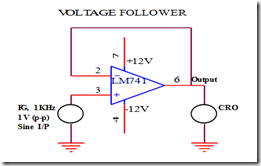### LIC–IC Fabrication (Unit 1) 2 Marks with answers

147404 - LINEAR INTEGRATED CIRCUITS

UNIT-I

IC FABRICATION AND CIRCUIT CONFIGURATION FOR LINEAR ICS

1. Mention the advantages of integrated circuits.

*Miniaturisation and hence increased equipment density.

*Cost reduction due to batch processing.

*Increased system reliability due to the elimination of soldered joints.

*Improved functional performance.

*Matched devices.

*Increased operating speeds.

*Reduction in power consumption.

2. Write down the various processes used to fabricate IC’s using silicon planar technology.

*Silicon wafer preparation.

* Epitaxial growth

*Oxidation.

*Photolithography.

*Diffusion.

*Ion implantation.

*Isolation.

*Metallisation.

*Assembly processing and packaging.

3. What is the purpose of oxidation?

*SiO2 is an extremely hard protective coating and is unaffected by almost all reagents.

*By selective etching of SiO2, diffusion of impurities through carefully defined windows can be accomplished to fabricate various components.

4. Why aluminum is preferred for metallization?

*It is a good conductor.

*it is easy to deposit aluminium films using vacuum deposition.

*It makes good mechanical bonds with silicon.

*It forms a low resistance contact.

5. What are the popular IC packages available?

a. Metal can package.

b. Dual-in-line package. c. Ceramic flat package.

6. Define an operational amplifier.

An operational amplifier is a direct-coupled, high gain amplifier consisting of one or more differential amplifier. By properly selecting the external components, it can be used to perform a variety of mathematical operations.

7. List out the ideal characteristics, and draw the equivalent diagram of an OP-AMP

* Open loop voltage gain is infinity.

*Input impedance is infinity.

*Output impedance is zero.

*Bandwidth is infinity.

*Zero offset.

8. Define Virtual ground property of an OP-AMP

A virtual ground is a ground which acts like a ground. It may not have physical connection to ground. This property of an ideal op-amp indicates that the inverting and non-inverting terminals of op-amp are at the same potentials. The non-inverting input is grounded for the inverting amplifier circuit. This means that the inverting input of the op-amp is also at ground potential.

9. Draw the voltage follower circuit of an OP-AMP10. Define the following parameters as applied to an op-amp:

i) Input bias current

Input bias current IB is the average of the currents that flow into the inverting and non-inverting input erminals of the op-amp.

i.e. IB = (IB1+IB2)/2

ii) Input offset current

The algebraic difference between the current into the inverting and non-inverting terminals is referred to as input offset current Iio. Mathematically it is represented as Iio = |IB - IB |

Where

I B+is the current into the non-inverting input terminals.

IB- is the current into the inverting input terminals.

iii) Input offset voltage

This is the voltage required to be amplified at the input for making output voltage to zero volts.

iv) C.M.R.R

The common mode rejection ratio (CMRR) can be defined as the ratio of differential gain to common mode gain.

v) P.S.R.R

Power Supply Rejection Ratio (PSRR) is the ability of an amplifier to maintain its output voltage as its

DC power-supply voltage is varied.

PSRR = (change in Vcc)/(change in Vout)

vi) slew rate

Slew rate can be defined as the maximum rate of change of output voltage of op-amp with respect to time. It is expressed as S = (dVo / dt) max in V/Sec.

Where slew rate S = 2П f Vm in V/Sec.

11. Why open loop op-amp configurations is not used in linear applications?

a. The open loop gain of the op-amp is very high. Therefore only the smaller signals having low frequency may be amplified accurately without distortion.

b. Open loop Voltage gain of the op-amp is not a constant voltage gain varies with changes in temperature and power supply as well as mass production techniques. This makes op-amp unsuitable for many linear applications

c. Bandwidth of most open loop op-amps is negligibly small or almost zero therefore op-amp is impractical in ac applications.

12. Determine the slew rate of the op-amp.

Slew rate can be defined as the maximum rate of change of output voltage of op-amp with respect to time. It is expressed as S = (dVo / dt) max in V/Sec.

Where slew rate S = 2П f Vm in V/Sec.

13. What is active load? Where it is used and why?

In circuit design, an active load is a circuit component made up of active devices, such as transistors, intended to present a high small-signal impedance yet not requiring a large DC voltage drop, as would occur if a large resistor were used instead. Such large AC load impedances may be desirable, for example, to increase the AC gain of some types of amplifier.

Most commonly the active load is the output part of a current mirror and is represented in an idealized manner as a current source. Usually, it is only a constant-current resistor that is a part of the whole current source including a constant voltage source as well